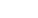How Cheenta works to ensure student success?
Explore the Back-Story

# Application of eigenvalue in degree 3 polynomial: ISI MMA 2018 Question 14# Understand the problem

[/et_pb_text][et_pb_tabs active_tab_background_color="#0c71c3" inactive_tab_background_color="#000000" _builder_version="3.27" tab_text_color="#ffffff" tab_font="||||||||" background_color="#ffffff" hover_enabled="0" _i="2" _address="0.1.0.2"][et_pb_tab title="Hint 0" _builder_version="3.22.4" _i="0" _address="0.1.0.2.0"]Do you really need a hint? Try it first!

[/et_pb_tab][et_pb_tab title="Hint 1" _builder_version="3.27" hover_enabled="0" _i="1" _address="0.1.0.2.1"]Let A be a 3 x 3 real matrix with trace 0 Now( 1+i) be an eigen value . (Eigenvalues are a special set of scalars associated with a linear system of equations (i.e., a matrix equation) that are sometimes also known as characteristic roots, characteristic values, proper values, or latent roots & Eigen vectors are In linear algebra,) (An eigenvector  or characteristic vector of a linear transformation is a non-zero vector that changes by only a scalar factor when that linear transformation is applied to it. More formally, if T is a linear transformation from a vector space V over a field F into itself and v is a vector in V that is not the zero vector, then v is an eigenvector of T if T(v) is a scalar multiple of v. This condition can be written as the equation ) [/et_pb_tab][et_pb_tab title="Hint 2" _builder_version="3.27" hover_enabled="0" _i="2" _address="0.1.0.2.2"]So ,( 1 + i) be the roots of the characteristic poly of A  Now A is a real matrix, char poly of A[Right!] Therefore ( 1 - i) is also a root of char poly of A[/et_pb_tab][et_pb_tab title="Hint 3" _builder_version="3.27" hover_enabled="0" _i="3" _address="0.1.0.2.3"]deg( char poly of A ) =3 So , it has two imaginary roots & one real root Let real root be r Note tr(A) = 0 => r + 1 +i +1 -i = 0 , => r = -2 Can you play with the determinants now ?[/et_pb_tab][et_pb_tab title="Hint 4" _builder_version="3.27" hover_enabled="0" _i="4" _address="0.1.0.2.4"]We know the determinant of A is the product of all eigen values  (-2)(1+i)(1-i) = detA => det(A) = -2[1 +1] = -4[/et_pb_tab][/et_pb_tabs][et_pb_text _builder_version="3.23.3" text_font="Raleway|300|||||||" text_text_color="#ffffff" header_font="Raleway|300|||||||" header_text_color="#e2e2e2" background_color="#0c71c3" border_radii="on|5px|5px|5px|5px" box_shadow_style="preset3" custom_margin="48px||48px" custom_padding="20px|20px|20px|20px" _i="3" _address="0.1.0.3"]

# Understand the problem

[/et_pb_text][et_pb_tabs active_tab_background_color="#0c71c3" inactive_tab_background_color="#000000" _builder_version="3.27" tab_text_color="#ffffff" tab_font="||||||||" background_color="#ffffff" hover_enabled="0" _i="2" _address="0.1.0.2"][et_pb_tab title="Hint 0" _builder_version="3.22.4" _i="0" _address="0.1.0.2.0"]Do you really need a hint? Try it first!

[/et_pb_tab][et_pb_tab title="Hint 1" _builder_version="3.27" hover_enabled="0" _i="1" _address="0.1.0.2.1"]Let A be a 3 x 3 real matrix with trace 0 Now( 1+i) be an eigen value . (Eigenvalues are a special set of scalars associated with a linear system of equations (i.e., a matrix equation) that are sometimes also known as characteristic roots, characteristic values, proper values, or latent roots & Eigen vectors are In linear algebra,) (An eigenvector  or characteristic vector of a linear transformation is a non-zero vector that changes by only a scalar factor when that linear transformation is applied to it. More formally, if T is a linear transformation from a vector space V over a field F into itself and v is a vector in V that is not the zero vector, then v is an eigenvector of T if T(v) is a scalar multiple of v. This condition can be written as the equation ) [/et_pb_tab][et_pb_tab title="Hint 2" _builder_version="3.27" hover_enabled="0" _i="2" _address="0.1.0.2.2"]So ,( 1 + i) be the roots of the characteristic poly of A  Now A is a real matrix, char poly of A[Right!] Therefore ( 1 - i) is also a root of char poly of A[/et_pb_tab][et_pb_tab title="Hint 3" _builder_version="3.27" hover_enabled="0" _i="3" _address="0.1.0.2.3"]deg( char poly of A ) =3 So , it has two imaginary roots & one real root Let real root be r Note tr(A) = 0 => r + 1 +i +1 -i = 0 , => r = -2 Can you play with the determinants now ?[/et_pb_tab][et_pb_tab title="Hint 4" _builder_version="3.27" hover_enabled="0" _i="4" _address="0.1.0.2.4"]We know the determinant of A is the product of all eigen values  (-2)(1+i)(1-i) = detA => det(A) = -2[1 +1] = -4[/et_pb_tab][/et_pb_tabs][et_pb_text _builder_version="3.23.3" text_font="Raleway|300|||||||" text_text_color="#ffffff" header_font="Raleway|300|||||||" header_text_color="#e2e2e2" background_color="#0c71c3" border_radii="on|5px|5px|5px|5px" box_shadow_style="preset3" custom_margin="48px||48px" custom_padding="20px|20px|20px|20px" _i="3" _address="0.1.0.3"]

# Similar Problems

This site uses Akismet to reduce spam. Learn how your comment data is processed.

### Knowledge Partner